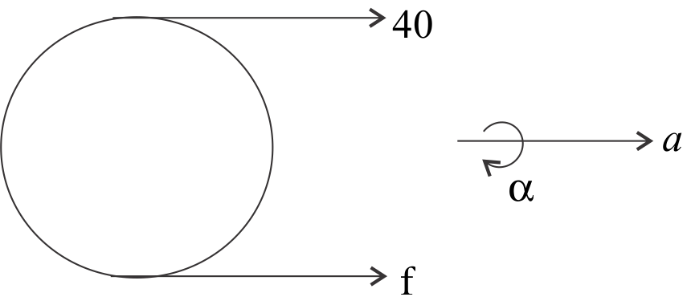Courses
Courses for Kids
Free study material
Offline Centres
MoreLast updated date: 06th Dec 2023
Total views: 345.3k
Views today: 7.45k

# A string is wound around a hollow cylinder of mass $5kg$ and radius $0.5m$ . If the string is now pulled with a horizontal force of $40N$ , and the cylinder is rolling without slipping on a horizontal surface (see figure), then the angular acceleration of the cylinder will be?(Neglect the mass and thickness of the string).A) $12rad{s^{ - 2}}$B) $16rad{s^{ - 2}}$C) $10rad{s^{ - 2}}$D) $20rad{s^{ - 2}}$Verified
345.3k+ views
Hint: Since, the force of $40N$ is acting on the cylinder in the right direction (as shown in diagram), then there must be some linear acceleration of the center of mass of the cylinder in the right direction because of the force acting on it. And this force is applied on the edge of the cylinder, then this will generate a torque in the cylinder and due to this torque, the cylinder will roll and hence will have some angular acceleration too.

Let linear acceleration in the direction of net force be a, angular acceleration be $\alpha$ .
Mass of cylinder is $m = 5kg$
Since, the force of $40N$ and force f is acting in same direction, so, equation for linear acceleration will be:
$40 + f = ma$
Replace $a = R\alpha$
$40 + f = mR\alpha$ -----(1)
Now, equation of torque –
$40 \times R - f \times R = m{R^2}\alpha \\ 40 - f = mR\alpha \\$
Let this be equation 2
Adding equation 2 with equation 1 and putting all given values, we get,
$80 = 2mR\alpha = 2 \times 5 \times 0.5 \times \alpha$
On solving this, we get angular acceleration as,
$\alpha = \dfrac{{80}}{5} = 16rad{s^{ - 2}}$
Hence angular acceleration of the cylinder will be $16rad{s^{ - 2}}$ .
So correct option is (B)

Note: We got equation 2 using relation between torque and force and using force balance. According to the given diagram, torque will be acting in clockwise direction and equation 1 we got using simple linear force balance in the right direction. So the cylinder will be rotating in clockwise direction as well as moving forward in the right direction simultaneously.